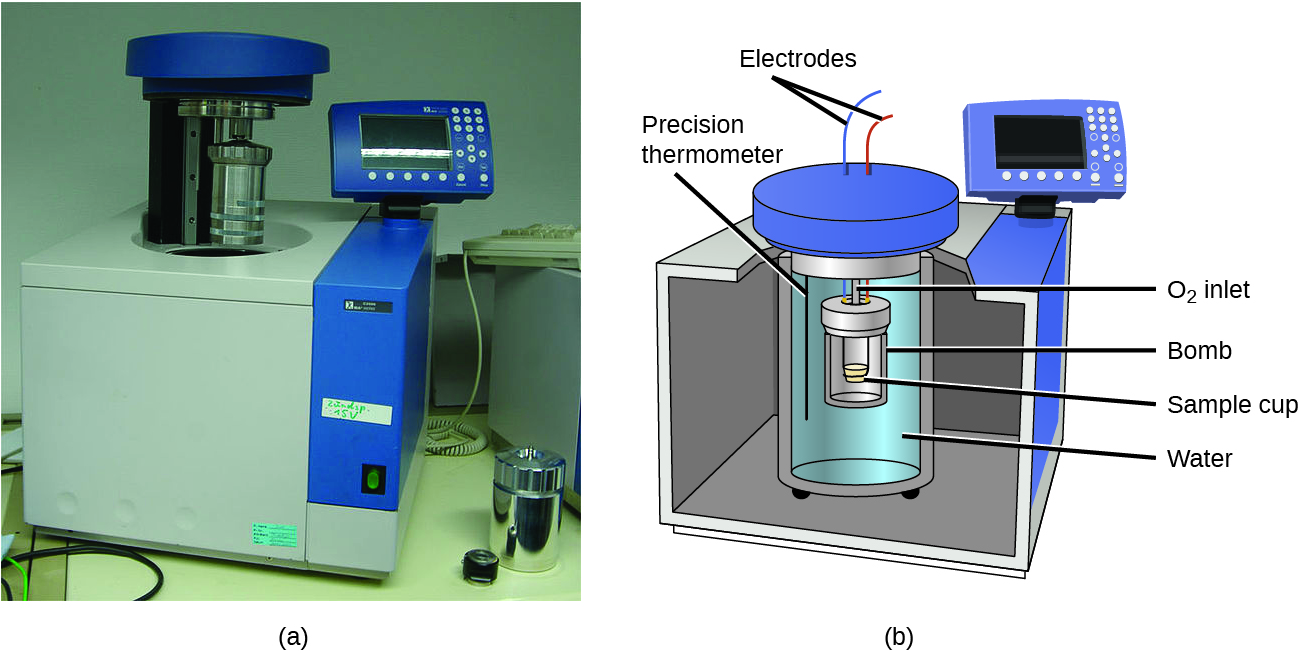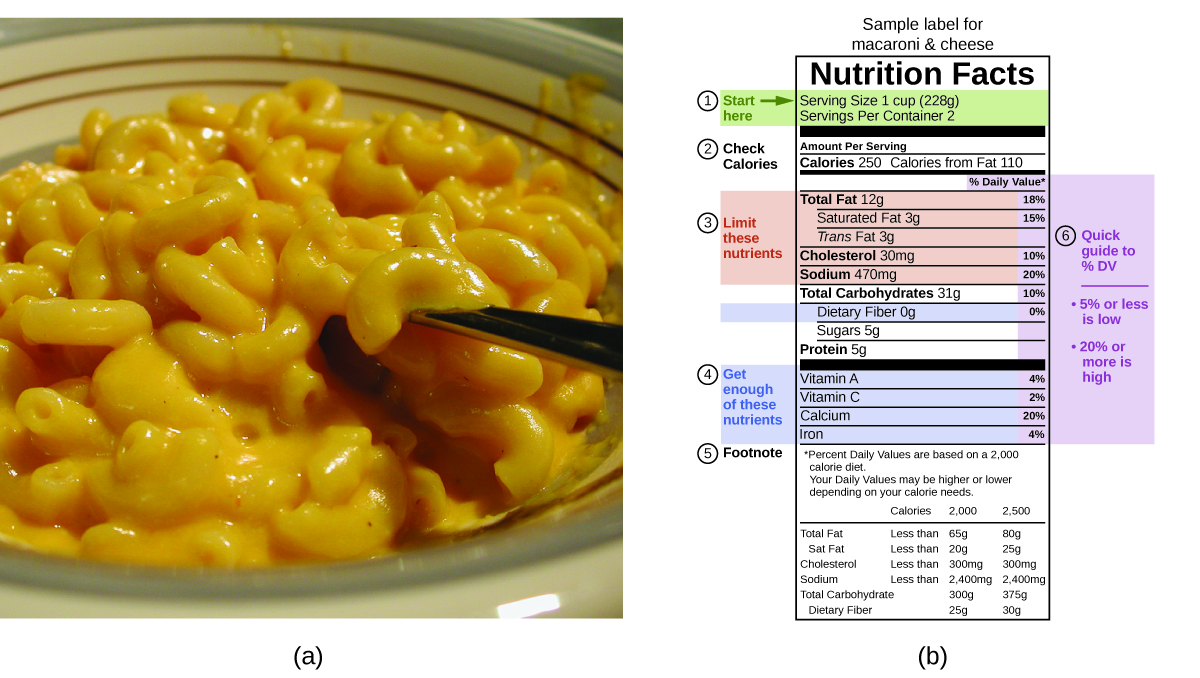# 5.4 Thermochemistry: calorimetry  (Page 6/14)

 Page 6 / 14(a) A bomb calorimeter is used to measure heat produced by reactions involving gaseous reactants or products, such as combustion. (b) The reactants are contained in the gas-tight “bomb,” which is submerged in water and surrounded by insulating materials. (credit a: modification of work by “Harbor1”/Wikimedia commons)

## Bomb calorimetry

When 3.12 g of glucose, C 6 H 12 O 6 , is burned in a bomb calorimeter, the temperature of the calorimeter increases from 23.8 °C to 35.6 °C. The calorimeter contains 775 g of water, and the bomb itself has a heat capacity of 893 J/°C. How much heat was produced by the combustion of the glucose sample?

## Solution

The combustion produces heat that is primarily absorbed by the water and the bomb. (The amounts of heat absorbed by the reaction products and the unreacted excess oxygen are relatively small and dealing with them is beyond the scope of this text. We will neglect them in our calculations.)

The heat produced by the reaction is absorbed by the water and the bomb:

$\begin{array}{l}{q}_{\text{rxn}}=-\left({q}_{\text{water}}+{q}_{\text{bomb}}\right)\\ =-\left[\left(4.184\phantom{\rule{0.2em}{0ex}}\text{J/g °C}\right)\phantom{\rule{0.2em}{0ex}}×\phantom{\rule{0.2em}{0ex}}\left(775\phantom{\rule{0.2em}{0ex}}\text{g}\right)\phantom{\rule{0.2em}{0ex}}×\phantom{\rule{0.2em}{0ex}}\left(35.6\phantom{\rule{0.2em}{0ex}}\text{°C}-23.8\phantom{\rule{0.2em}{0ex}}\text{°C}\right)+893\phantom{\rule{0.2em}{0ex}}\text{J/°C}\phantom{\rule{0.2em}{0ex}}×\phantom{\rule{0.2em}{0ex}}\left(35.6\phantom{\rule{0.2em}{0ex}}\text{°C}-23.8\phantom{\rule{0.2em}{0ex}}\text{°C}\right)\right]\\ =-\left(38,300\phantom{\rule{0.2em}{0ex}}\text{J}+10,500\phantom{\rule{0.2em}{0ex}}\text{J}\right)\\ =\text{−48,800 J}=\text{−48.8 kJ}\end{array}$

This reaction released 48.7 kJ of heat when 3.12 g of glucose was burned.

## Check your learning

When 0.963 g of benzene, C 6 H 6 , is burned in a bomb calorimeter, the temperature of the calorimeter increases by 8.39 °C. The bomb has a heat capacity of 784 J/°C and is submerged in 925 mL of water. How much heat was produced by the combustion of the glucose sample?

## Answer:

39.0 kJ

Since the first one was constructed in 1899, 35 calorimeters have been built to measure the heat produced by a living person. Francis D. Reardon et al. “The Snellen human calorimeter revisited, re-engineered and upgraded: Design and performance characteristics.” Medical and Biological Engineering and Computing 8 (2006)721–28, http://link.springer.com/article/10.1007/s11517-006-0086-5. These whole-body calorimeters of various designs are large enough to hold an individual human being. More recently, whole-room calorimeters allow for relatively normal activities to be performed, and these calorimeters generate data that more closely reflect the real world. These calorimeters are used to measure the metabolism of individuals under different environmental conditions, different dietary regimes, and with different health conditions, such as diabetes. In humans, metabolism is typically measured in Calories per day. A nutritional calorie (Calorie)    is the energy unit used to quantify the amount of energy derived from the metabolism of foods; one Calorie is equal to 1000 calories (1 kcal), the amount of energy needed to heat 1 kg of water by 1 °C.

## Measuring nutritional calories

In your day-to-day life, you may be more familiar with energy being given in Calories, or nutritional calories, which are used to quantify the amount of energy in foods. One calorie (cal) = exactly 4.184 joules, and one Calorie (note the capitalization) = 1000 cal, or 1 kcal. (This is approximately the amount of energy needed to heat 1 kg of water by 1 °C.)

The macronutrients in food are proteins, carbohydrates, and fats or oils. Proteins provide about 4 Calories per gram, carbohydrates also provide about 4 Calories per gram, and fats and oils provide about 9 Calories/g. Nutritional labels on food packages show the caloric content of one serving of the food, as well as the breakdown into Calories from each of the three macronutrients ( [link] ).(a) Macaroni and cheese contain energy in the form of the macronutrients in the food. (b) The food’s nutritional information is shown on the package label. In the US, the energy content is given in Calories (per serving); the rest of the world usually uses kilojoules. (credit a: modification of work by “Rex Roof”/Flickr)

For the example shown in (b), the total energy per 228-g portion is calculated by:

$\left(5\phantom{\rule{0.2em}{0ex}}\text{g protein}\phantom{\rule{0.2em}{0ex}}×\phantom{\rule{0.2em}{0ex}}4\phantom{\rule{0.2em}{0ex}}\text{Calories/g}\right)+\left(31\phantom{\rule{0.2em}{0ex}}\text{g carb}\phantom{\rule{0.2em}{0ex}}×\phantom{\rule{0.2em}{0ex}}4\phantom{\rule{0.2em}{0ex}}\text{Calories/g}\right)+\left(12\phantom{\rule{0.2em}{0ex}}\text{g fat}\phantom{\rule{0.2em}{0ex}}×\phantom{\rule{0.2em}{0ex}}9\phantom{\rule{0.2em}{0ex}}\text{Calories/g}\right)=252\phantom{\rule{0.2em}{0ex}}\text{Calories}$

So, you can use food labels to count your Calories. But where do the values come from? And how accurate are they? The caloric content of foods can be determined by using bomb calorimetry; that is, by burning the food and measuring the energy it contains. A sample of food is weighed, mixed in a blender, freeze-dried, ground into powder, and formed into a pellet. The pellet is burned inside a bomb calorimeter, and the measured temperature change is converted into energy per gram of food.

Today, the caloric content on food labels is derived using a method called the Atwater system that uses the average caloric content of the different chemical constituents of food, protein, carbohydrate, and fats. The average amounts are those given in the equation and are derived from the various results given by bomb calorimetry of whole foods. The carbohydrate amount is discounted a certain amount for the fiber content, which is indigestible carbohydrate. To determine the energy content of a food, the quantities of carbohydrate, protein, and fat are each multiplied by the average Calories per gram for each and the products summed to obtain the total energy.

#### Questions & Answers

what is math number
Tric Reply
x-2y+3z=-3 2x-y+z=7 -x+3y-z=6
Sidiki Reply
Need help solving this problem (2/7)^-2
Simone Reply
x+2y-z=7
Sidiki
what is the coefficient of -4×
Mehri Reply
-1
Shedrak
the operation * is x * y =x + y/ 1+(x × y) show if the operation is commutative if x × y is not equal to -1
Alfred Reply
An investment account was opened with an initial deposit of $9,600 and earns 7.4% interest, compounded continuously. How much will the account be worth after 15 years? Kala Reply lim x to infinity e^1-e^-1/log(1+x) given eccentricity and a point find the equiation Moses Reply 12, 17, 22.... 25th term Alexandra Reply 12, 17, 22.... 25th term Akash College algebra is really hard? Shirleen Reply Absolutely, for me. My problems with math started in First grade...involving a nun Sister Anastasia, bad vision, talking & getting expelled from Catholic school. When it comes to math I just can't focus and all I can hear is our family silverware banging and clanging on the pink Formica table. Carole I'm 13 and I understand it great AJ I am 1 year old but I can do it! 1+1=2 proof very hard for me though. Atone hi Adu Not really they are just easy concepts which can be understood if you have great basics. I am 14 I understood them easily. Vedant find the 15th term of the geometric sequince whose first is 18 and last term of 387 Jerwin Reply I know this work salma The given of f(x=x-2. then what is the value of this f(3) 5f(x+1) virgelyn Reply hmm well what is the answer Abhi If f(x) = x-2 then, f(3) when 5f(x+1) 5((3-2)+1) 5(1+1) 5(2) 10 Augustine how do they get the third part x = (32)5/4 kinnecy Reply make 5/4 into a mixed number, make that a decimal, and then multiply 32 by the decimal 5/4 turns out to be AJ how Sheref can someone help me with some logarithmic and exponential equations. Jeffrey Reply sure. what is your question? ninjadapaul 20/(×-6^2) Salomon okay, so you have 6 raised to the power of 2. what is that part of your answer ninjadapaul I don't understand what the A with approx sign and the boxed x mean ninjadapaul it think it's written 20/(X-6)^2 so it's 20 divided by X-6 squared Salomon I'm not sure why it wrote it the other way Salomon I got X =-6 Salomon ok. so take the square root of both sides, now you have plus or minus the square root of 20= x-6 ninjadapaul oops. ignore that. ninjadapaul so you not have an equal sign anywhere in the original equation? ninjadapaul hmm Abhi is it a question of log Abhi 🤔. Abhi I rally confuse this number And equations too I need exactly help salma But this is not salma it's Faiza live in lousvile Ky I garbage this so I am going collage with JCTC that the of the collage thank you my friends salma Commplementary angles Idrissa Reply hello Sherica im all ears I need to learn Sherica right! what he said ⤴⤴⤴ Tamia hii Uday hi salma hi Ayuba Hello opoku hi Ali greetings from Iran Ali salut. from Algeria Bach hi Nharnhar A soccer field is a rectangle 130 meters wide and 110 meters long. The coach asks players to run from one corner to the other corner diagonally across. What is that distance, to the nearest tenths place. Kimberly Reply Jeannette has$5 and \$10 bills in her wallet. The number of fives is three more than six times the number of tens. Let t represent the number of tens. Write an expression for the number of fives.
August Reply
What is the expressiin for seven less than four times the number of nickels
Leonardo Reply
How do i figure this problem out.
how do you translate this in Algebraic Expressions
linda Reply
why surface tension is zero at critical temperature
Shanjida
I think if critical temperature denote high temperature then a liquid stats boils that time the water stats to evaporate so some moles of h2o to up and due to high temp the bonding break they have low density so it can be a reason
s.
Need to simplify the expresin. 3/7 (x+y)-1/7 (x-1)=
Crystal Reply
. After 3 months on a diet, Lisa had lost 12% of her original weight. She lost 21 pounds. What was Lisa's original weight?
Chris Reply
how do you find theWhat are the wavelengths and energies per photon of two lines
caroline Reply
The eyes of some reptiles are sensitive to 850 nm light. If the minimum energy to trigger the receptor at this wavelength is 3.15 x 10-14 J, what is the minimum number of 850 nm photons that must hit the receptor in order for it to be triggered?
razzyd Reply
A teaspoon of the carbohydrate sucrose contains 16 calories, what is the mass of one teaspoo of sucrose if the average number of calories for carbohydrate is 4.1 calories/g?
ifunanya Reply
4. On the basis of dipole moments and/or hydrogen bonding, explain in a qualitative way the differences in the boiling points of acetone (56.2 °C) and 1-propanol (97.4 °C), which have similar molar masses
Kyndall Reply
Calculate the bond order for an ion with this configuration: (?2s)2(??2s)2(?2px)2(?2py,?2pz)4(??2py,??2pz)3
Gabe Reply
Which of the following will increase the percent of HF that is converted to the fluoride ion in water? (a) addition of NaOH (b) addition of HCl (c) addition of NaF
Tarun Reply

### Read also:

#### Get the best Algebra and trigonometry course in your pocket!

Source:  OpenStax, Ut austin - principles of chemistry. OpenStax CNX. Mar 31, 2016 Download for free at http://legacy.cnx.org/content/col11830/1.13
Google Play and the Google Play logo are trademarks of Google Inc.

Notification Switch

Would you like to follow the 'Ut austin - principles of chemistry' conversation and receive update notifications?ByBy